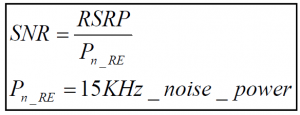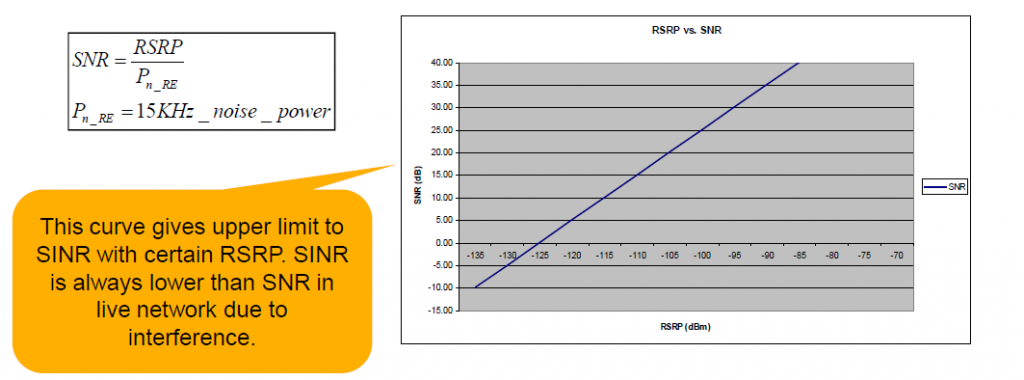# RSRP and RSRQ Measurement in LTE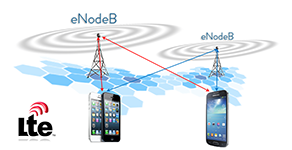RSRP and RSRQ are key measures of signal level and quality for modern LTE networks. In cellular networks, when a mobile moves from cell to cell and performs cell selection/reselection and handover, it has to measure the signal strength/quality of the neighbor cells.
In LTE network, a UE measures two parameters on reference signal: RSRP (Reference Signal Received Power) and RSRQ (Reference Signal Received Quality).

In LTE network, a UE measures two parameters on reference signal:

RSSI – Received Signal Strength Indicator:The carrier RSSI (Receive Strength Signal Indicator) measures the average total received power observed only in OFDM symbols containing reference symbols for antenna port 0 (i.e., OFDM symbol 0 & 4 in a slot) in the measurement bandwidth over N resource blocks.

The total received power of the carrier RSSI includes the power from co-channel serving & non-serving cells, adjacent channel interference, thermal noise, etc. Total measured over 12-subcarriers including RS from Serving Cell, Traffic in the Serving Cell

RSRP – Reference Signal Received Power: RSRP is a RSSI type of measurement, as follows there are some definition of it and some details as well.
It is the power of the LTE Reference Signals spread over the full bandwidth and narrowband.
A minimum of -20 dB SINR (of the S-Synch channel) is needed to detect RSRP/RSRQ

RSRQ – Reference Signal Received Quality: Quality considering also RSSI and the number of used Resource Blocks (N) RSRQ = (N * RSRP) / RSSI measured over the same bandwidth. RSRQ is a C/I type of measurement and it indicates the quality of the received reference signal. The RSRQ measurement provides additional information when RSRP is not sufficient to make a reliable handover or cell reselection decision.

In the procedure of handover, the LTE specification provides the flexibility of using RSRP, RSRQ, or both.

It must to be measured over the same bandwidth:

• Narrowband N = 62 Sub Carriers (6 Resource Blocks)
• Wideband N = full bandwidth (up to 100 Resource Blocks / 20 MHz)

RSRP 3GPP Definition

Reference Signal Received Power (RSRP), is defined as the linear average over the power contributions (in [W]) of the resource elements that carry cell-specific reference signals within the considered measurement frequency bandwidth.
For RSRP determination the cell-specific reference signals R0 according TS 36.211  shall be used. If the UE can reliably detect that R1 is available it may use R1 in addition to R0 to determine RSRP.
The reference point for the RSRP shall be the antenna connector of the UE.

Or, even better, Reference Signal Received Quality is defined as the ratio N×RSRP/(E-UTRA carrier RSSI), where N is the number of RB’s of the E-UTRA carrier RSSI measurement bandwidth. The measurements in the numerator and denominator shall be made over the same set of resource blocks.

If receiver diversity is in use by the UE, the reported value shall not be lower than the corresponding RSRP of any of the individual diversity branches.

Applicable for: RRC_IDLE intra-frequency, RRC_IDLE inter-frequency, RRC_CONNECTED intra-frequency, RRC_CONNECTED inter-frequency

Note1: The number of resource elements within the considered measurement frequency bandwidth and within the measurement period that are used by the UE to determine RSRP is left up to the UE implementation with the limitation that corresponding measurement accuracy requirements have to be fulfilled.

Note 2: The power per resource element is determined from the energy received during the useful part of the symbol, excluding the CP.

## RSRP

In other words RSRP (Reference Signal Receive Power) is the average power of Resource Elements (RE) that carry cell specific Reference Signals (RS) over the entire bandwidth, so RSRP is only measured in the symbols carrying RS.

• RSRP is the average received power of a single RS resource element.
• UE measures the power of multiple resource elements used to transfer the reference signal but then takes an average of them rather than summing them.
• Reporting range -44…-140 dBm

RSRP does a better job of measuring signal power from a specific sector while potentially excluding noise and interference from other sectors.

RSRP levels for usable signal typically range from about -75 dBm close in to an LTE cell site to -120 dBm at the edge of LTE coverage.

#### RSRP mapping 3GPP TS 36.133 V8.9.0 (2010-03)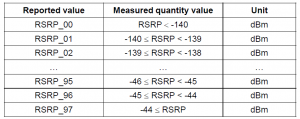The reporting range of RSRP is defined from -140 dBm to – 44 dBm with 1 dB resolution.

The mapping of measured quantity is defined in the table.

RSRQ 3GPP DefinitionReference Signal Received Quality (RSRQ) is defined as the ratio N×RSRP/(E-UTRA carrier RSSI), where N is the number of RB’s of the E-UTRA carrier RSSI measurement bandwidth. The measurements in the numerator and denominator shall be made over the same set of resource blocks.

E-UTRA Carrier Received Signal Strength Indicator (RSSI), comprises the linear average of the total received power (in [W]) observed only in OFDM symbols containing reference symbols for antenna port 0, in the measurement bandwidth, over N number of resource blocks by the UE from all sources, including co-channel serving and non-serving cells, adjacent channel interference, thermal noise etc.

The reference point for the RSRQ shall be the antenna connector of the UE. If receiver diversity is in use by the UE, the reported value shall not be lower than the corresponding RSRQ of any of the individual diversity branches.

Applicable for: RRC_CONNECTED intra-frequency, RRC_CONNECTED inter-frequency

## RSRQ

In formula:

#### RSRQ = N x RSRP / RSSI

•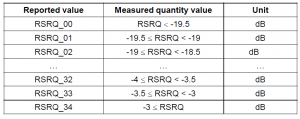N is the number of Physical Resource Blocks (PRBs) over which the RSSI is measured, typically equal to system bandwidth
• RSSI is pure wide band power measurement, including intracell power, interference and noise
• The reporting range of RSRQ is defined from -3…-19.5dB

#### Reference Signals recap: OFDMA Channel Estimation

In simple terms the Reference Signal (RS) is mapped to Resource Elements (RE). This mapping follows a specific pattern (see to below).

So at any point in time the UE will measure all the REs that carry the RS and average the measurements to obtain an RSRP reading.

• Channel estimation in LTE is based on reference signals (like CPICH functionality in WCDMA)
• Reference signals position in time domain is fixed (0 and 4 for Type 1 Frame) whereas in frequency domain it depends on the Cell ID
• In case more than one antenna is used (e.g. MIMO) the Resource elements allocated to reference signals on one antenna are DTX on the other antennas
• Reference signals are modulated to identify the cell to which they belong.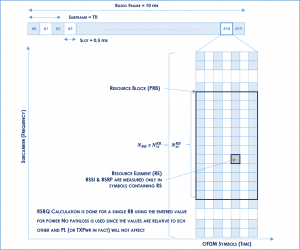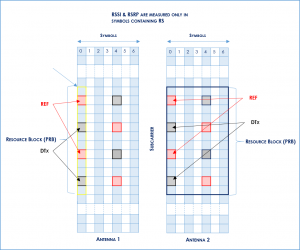RSSI (Received Signal Strength Indicator) is a parameter which provides information about total received wide-band power (measure in all symbols) including all interference and thermal noise.

RSSI is not reported to eNodeB by UE. It can simply be computed from RSRQ and RSRP that are, instead, reported by UE.

### RSSI = wideband power = noise + serving cell power + interference power

So, without noise and interference, we have that 100% DL PRB activity: RSSI=12*N*RSRP

Where:

• RSRP is the received power of 1 RE (3GPP definition) average of power levels received across all Reference Signal symbols within the considered measurement frequency bandwidth
• RSSI is measured over the entire bandwidth
• N: number of RBs across the RSSI is measured and depends on the BW

Based on the above, under full load and high:

### SNR: RSRP (dBm)= RSSI (dBm) -10*log (12*N)

So we have:

#### RSRQ = RSRP / (RSSI/N)

• N = Number of PRBs (Physical Resource Blocks)
• RSSI = noise + serving cell power + interference power during RS symbol
• So we have that RSRQ depends on serving cell power and the number of Tx antennas

#### Impact of serving cell power to RSRQ:

Example for noise limited case (no interference): If all resource elements are active and are transmitted with equal power, then

#### RSRQ = N / 20N = -13 dB for 2Tx taking DTX into account

(because RSRP is measured over 1 resource element and RSSI per resource block is measured over 12 resource elements).

Remember that RSSI is only measured at those symbol times during which RS REs are transmitted – We do not have to take into the count DTx!!!

So, when there is no traffic, and assuming only the reference symbols are transmitted (there are 2 of them within the same symbol of a resource block) from a single Tx antenna then the RSSI is generated by only the 2 reference symbols so the result becomes

#### RSRQ = -6dB for 2Tx

SINR DefinitionSINR is the reference value used in the system simulation and can be defined:

1. Wide band SINR
2. SINR for a specific subcarriers (or for a specific resource elements)

### SNR vs. RSRP

RSRP is measured for a single subcarrier, noisepower for 15KHz= -125.2dBm

1. Noise figure = 7 dB
2. Temperature = 290 K

Assumption: RSRP doesn’t contain noise power